# Winsorization

• Last Updated : 30 May, 2021

Winsorization is the process of replacing the extreme values of statistical data in order to limit the effect of the outliers on the calculations or the results obtained by using that data. The mean value calculated after such replacement of the extreme values is called winsorized mean.

For example, 90% winsorization means the replacement of the top 5% and bottom 5% of the data. The top 5% of the data is replaced by the value of the data at the 95th percentile and the value of the bottom 5% of the data is replaced by the value of the data at the 5th percentile.

Input:

• A numeric array whose values at the upper end and the lower end are to be winsorized.
• The first argument of the tuple is the percentage of values at the lower end which are to be winsorized.
• The second argument of the tuple is the percentage of values at the upper end which are to be winsorized.

Output:

A numeric array whose values at the upper end and at the lower end are winsorized as defined by the user.

Example #1:

## Python3

 `# Libraries to be imported ` `import` `numpy as np ` `import` `matplotlib.pyplot as plt ` `import` `random ` `from` `scipy.stats.mstats ``import` `winsorize`

Let us see an example where outliers are present on both the upper end and the lower end of the data.

## Python3

 `# Creating an array with 100 random values ` `array ``=` `[np.random.randint(``100``) ``for` `i ``in` `range``(``100``)] ` ` `  `# Creating outliers ` `# Here, the values which are selected for creating outliers  ` `# are appended so that same outliers are not created again. ` `AlreadySelected ``=` `[] ` `i ``=` `0` ` `  `# Creating 5 outliers on the lower end ` `while` `(i < ``5``): ` `    ``x ``=` `np.random.choice(array)  ``# Randomly selecting a value from the array ` `    ``y ``=` `x ``-` `mean``*``3` `    ``array ``=` `np.append(array, y) ` `    ``if` `(x ``not` `in` `already_selected): ` `        ``AlreadySelected.append(y) ` ` `  `        ``i ``+``=` `1` ` `  `    ``else``: ` `        ``continue` ` `  `# Creating 5 outliers on the upper end ` `i ``=` `0` `while` `(i < ``5``): ` `    ``x ``=` `np.random.choice(array)  ``# Randomly selecting a value from the array ` `    ``y ``=` `x ``+` `mean``*``4` `    ``array ``=` `np.append(array, y) ` `    ``if` `(x ``not` `in` `already_selected): ` `        ``AlreadySelected.append(y) ` ` `  `        ``i ``+``=` `1` ` `  `    ``else``: ` `        ``continue` ` `  `std ``=` `np.std(array)  ``# Storing the standard deviation of the array ` `mean ``=` `np.mean(array)  ``# Storing the mean of the array ` ` `  `plt.boxplot(array) ` `plt.title(``'Array with Outliers'``) ` `plt.show() `

Output: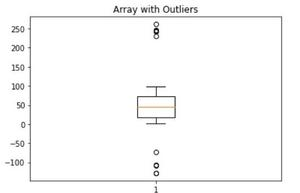## Python3

 `print``(mean) ``# mean of the numeric array with outliers`

Output: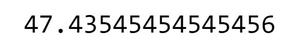Now, we winsorize the array by 10% i.e. we winsorize 5% of the highest values and 5% of the lowest value of the array:

## Python3

 `WinsorizedArray ``=` `winsorize(array,(``0.05``,``0.05``)) ` ` `  `plt.boxplot(WinsorizedArray) ` `plt.title(``'Winsorized array'``) ` `plt.show()`

Output: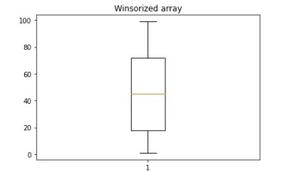## Python3

 `WinsorizedMean ``=` `np.mean(WinsorizedArray) ` `print``(WinsorizedMean)`

Output:In this case, there is only a slight change in the mean value of the data.

Now, let us see an example where outliers are present only at one end of the data.

## Python3

 `# Creating another array with 100 random values ` `array2 ``=` `[np.random.randint(``100``) ``for` `i ``in` `range``(``100``)]  ` `std ``=` `np.std(array2) ` `mean ``=` `np.mean(array2) ` `AlreadySelected ``=` `[] ` `# Creating outliers on the upper end ` `i ``=` `0`  `while` `(i<``5``): ` `    ``x ``=` `np.random.choice(array2) ``# Randomly selecting a value from the array ` `    ``y ``=` `x ``+` `mean``*``4` `    ``array2 ``=` `np.append(array2,y) ` `    ``if` `(x ``not` `in` `AlreadySelected): ` `        ``AlreadySelected.append(y) ` ` `  `        ``i``+``=``1` `         `  `    ``else``: ` `        ``continue` `         `  `plt.boxplot(array2) ` `plt.title(``'Array with outliers'``) ` `plt.show()`

Output: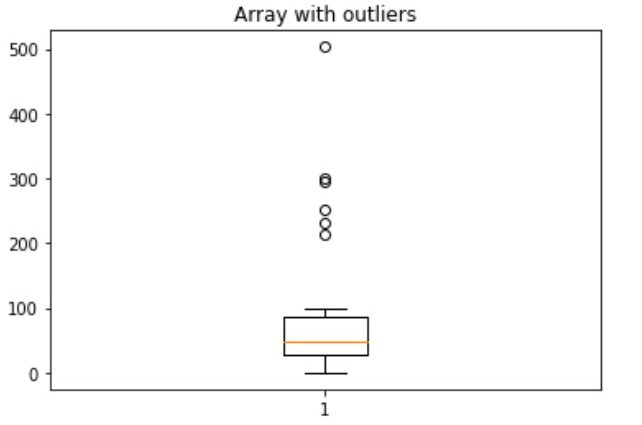## Python3

 `OutlierArray2Mean ``=` `np.mean(array2) ` `print``(OutlierArray2Mean)`

Output: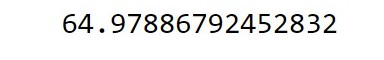## Python3

 `WinsorizedArray2 ``=` `winsorize(array2,(``0.1``,``0.1``)) ` `# In this case, the lower 10% values of  ` `# the data will have their values set equal to the value of the data point at  ` `#the 10th percentile. ` ` `  `plt.boxplot(WinsorizedArray2) ` `plt.show() ` ` `  `WinsorizedArray2Mean ``=` `np.mean(WinsorizedArray2) `

Output: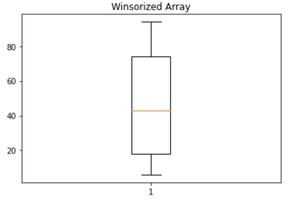## Python3

 `WinsorizedArray2Mean ``=` `np.mean(WinsorizedArray2) ` `print``(WinsorizedArray2Mean)`

Output: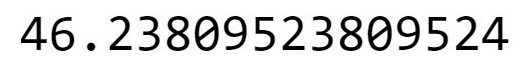In this case, there is a significant difference in the mean value.

My Personal Notes arrow_drop_up
Recommended Articles
Page :